Processing ......FreeComputerBooks.com Links to Free Computer, Mathematics, Technical Books all over the World

Probability: Theory and Examples
Top Free Data Science Books 🌠 - 100% Free or Open Source!
• Title: Probability: Theory and Examples
• Author(s) Rick Durrett
• Publisher: Cambridge University Press; 5th Ed. (May 30, 2019); eBook (Draft, Version 5, January 11, 2019)
• Permission: The PDF Draft is post by the author.
• Hardcover 430 pages
• eBook PDF (490 pages)
• Language: English
• ISBN-10/ASIN: 1108473687
• ISBN-13: 978-1108473682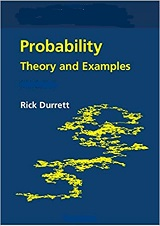Book Description

This book is a classic introduction to probability theory covering laws of large numbers, central limit theorems, random walks, martingales, Markov chains, ergodic theorems, and Brownian motion. It is a comprehensive treatment concentrating on the results that are the most useful for applications. Its philosophy is that the best way to learn probability is to see it in action, so there are 200 examples and 450 problems.

This book is also an excellent resource. Several interesting and concrete examples are presented throughout the textbook, which will help novices obtain a better understanding of the fundamentals of probability theory

The best feature of the book is its selection of examples. The author has done an extraordinary job in showing not simply what the presented theorems can be used for, but also what they cannot be used for.

The new edition of this lively but rigorous introduction to measure theoretic probability theory, designed for use in a graduate course, contains a new chapter on multidimensional Brownian motion and its relationship to partial differential equations (PDEs), a topic that is finding new applications.

• Rick Durrett received his Ph.D. in Operations Research from Stanford University in 1976. After nine years at UCLA and twenty-five at Cornell University, he moved to Duke University in 2010, where he is a Professor of Mathematics. He is the author of 8 books and more than 170 journal articles on a wide variety of topics, and he has supervised more than 40 Ph.D. students. He is a member of the National Academy of Science and the American Academy of Arts and Sciences and a Fellow of the Institute of Mathematical Statistics.
Reviews, Ratings, and Recommendations: Related Book Categories: Read and Download Links:Similar Books:
•Probability Theory: The Logic of Science (E. T. Jaynes)

Going beyond the conventional mathematics of probability theory, this study views the subject in a wider context. It discusses new results, along with applications of probability theory to a variety of problems.

•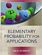Elementary Probability for Applications (Rick Durrett)

This clear and lively introduction to probability theory concentrates on the results that are the most useful for applications, including combinatorial probability and Markov chains. Concise and focused, it is designed for students familiar with basic calculus.

•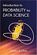Introduction to Probability for Data Science (Stanley Chan)

This book is an introductory textbook in undergraduate probability in the context of data science to emphasize the inseparability between data (computing) and probability (theory) in our time, with examples in both MATLAB and Python.

•Introduction to Probability, Statistics, and Random Processes

This book introduces students to probability, statistics, and stochastic processes. It provides a clear and intuitive approach to these topics while maintaining mathematical accuracy. The book contains a large number of solved exercises.

•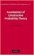Foundations of Constructive Probability Theory (Yuen-Kwok Chan)

This book provides a systematic and general theory of probability within the framework of Constructive Mathematics. It can serve as a parallel introduction into constructive mathematics and rigorous probability theory.

•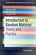Introduction to Random Matrices: Theory and Practice

This is a book for absolute beginners. The aim is to provide a truly accessible introductory account of Random Matrix theory. Most chapters are accompanied by MATLAB codes to guide readers through the numerical check of most analytical results.

•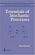Essentials of Stochastic Processes (Rick Durrett)

Stochastic processes have become important for many fields, including mathematical finance and engineering. Written by one of the worlds leading probabilists, this book presents recent results previously available only in specialized monographs.

•Introduction to Probability (Charles M. Grinstead, et al)

The book is a beautiful introduction to probability theory at the beginning level. The book contains a lot of examples and an easy development of theory without any sacrifice of rigor, keeping the abstraction to a minimal level.

•Probability Theory: The Logic of Science (E. T. Jaynes)

Going beyond the conventional mathematics of probability theory, this study views the subject in a wider context. It discusses new results, along with applications of probability theory to a variety of problems.

•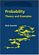Probability: Theory and Examples (Rick Durrett)

This lively introduction to measure-theoretic probability theory covers laws of large numbers, central limit theorems, random walks, martingales, Markov chains, ergodic theorems, and Brownian motion.

•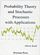Probability Theory and Stochastic Processes with Applications

This book provides an introduction to probability theory and discrete and continuous stochastic processes and its applications. It has a unique approach that provides a broad and wide introduction into the fascinating area of probability theory.

Book Categories
 :All CategoriesRecent BooksMiscellaneous BooksComputer LanguagesComputer ScienceData Science/DatabasesElectrical EngineeringJava and Java EE (J2EE)Linux and UnixMathematicsMicrosoft and .NETMobile ComputingNetworking and CommunicationsSoftware EngineeringSpecial TopicsWeb Programming
Other Categories